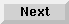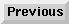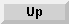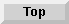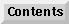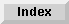1.1.2 Differences between LOOP and DO

# 1.1.2.3 Example 3

```;;; These functions sum the elements of an array. Since the Loop
;;; Facility supports iteration over arrays, the code is simple.
(defun sum-array-loop (array)               ; Use Loop Facility
(loop for a being the elements of array   ; constructs.
sum a))

(defun sum-array-do (array)                 ; Use the DO macro.
(do ((index 0 (1+ index))
(sum 0))
((= index (length array)) sum)
(incf sum (aref array index))))

```

The Loop Facility - 9 SEP 1996Generated with Harlequin WebMaker